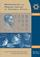Középiskolai Matematikai és Fizikai Lapok
Informatika rovattal
 Már regisztráltál? Új vendég vagy?# Angol nyelvű szám, 2002. december

Előző oldalTartalomjegyzékKövetkező oldalMEGRENDELŐLAP

## Solutions of problems B

B. 3429. Let $\displaystyle q=\frac{1+\sqrt{5}}{2}$, and let $\displaystyle f\colon\mathbb{N}\to\mathbb{N}$ be a function, such that for all positive integers n,

$\displaystyle \big|f(n)-qn\big|<\frac{1}{q}.$

Prove that f(f(n))= f(n)+n.

Solution. As $\displaystyle \frac{1}{q$\big|f(0)\big|">, obviously f(0)=0. For any other n, the value of f is a positive integer, otherwise f(n)=0<n would imply $\displaystyle \frac{1}{q$\big|f(n)-qn\big|=|-qn|"> and hence $\displaystyle n<\frac{1}{q^2}<1$, which is impossible. It is easy to check, furthermore, that $\displaystyle q(q-1)=\frac{\sqrt{5}+1}{2}\cdot\frac{\sqrt{5}-1}{2}=1$. Thus for any natural number n,

|f(f(n))- f(n)-n| = |f (f(n))- qf(n)+(q-1) f(n)- q(q-1)n| =
= |f (f(n))- qf(n)+ (q-1) (f(n)-qn)|.

As the inequality |a+b|$\displaystyle \le$|a|+|b| holds for all real numbers a, b, the above absolute value can be at most

|f (f(n))-qf(n)|+ | (q-1) (f(n)-qn)|= |f(f(n))-qf(n)|+ (q-1) |f(n)-qn|,

which is less than $\displaystyle \frac{1}{q}+(q-1)\frac{1}{q}=1$ according to the condition. Hence

|f(f(n))- f(n)-n|<1,

which can only happen if f(f(n))- f(n)-n=0, as f(f(n)), f(n) and n are integers.

G. Bóka, Szolnok